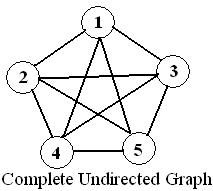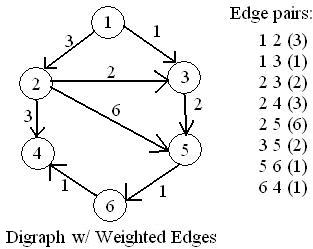# Lab 9: Shortest Path: Dijkstra's Algorithm

## Background

### Graphs p. 339

#### Definitions

• A graph G=(V, E) consists of a set of vertices, V, and a set of edges, E.
• Each edge (a.k.a. arc) is a pair (v, w) where v, w ∈ V. If the pair is ordered, then the graph is a directed graph (a.k.a. digraph).
• A vertex w is adjacent to v if and only if (v, w) ∈ E.
• An edge may have an assigned weight or cost.
• A path in a graph is a sequence of vertices w1, w2, w3, . . . , wN such that (wi, wi+1) ∈ E for 1<=i<N. The length of the path is the number of edges or N-1.• A cycle in a directed graph is a path of length at least 1 such that w1=wN.
• A directed graph is acyclic is it has no cycles. Such a graph is called a directed acyclic graphic or a DAG.
• An undirected graph is connected if there is a path from every vertex to every other vertex.
• A directed graph with this property is called strongly connected. If the directed would be considered connected if it were not directed, it is called weakly connected.
• A complete graph is a graph in which there is an edge between every pair of vertices.

#### Representations of Graphs

If each vertex in the graph is numbered, there are two common ways to represent graphs:
1. Adjacency matrix: a 2D array of boolean values. If there is an edge (u, v), then element [u][v] is true. If it is a weighted graph, then the matrix will hold the weights of the edges instead of boolean values. Unfortunately, the adjacency matrix requires |V|2 space to store the graph. If the graph has only a few edges, this is expensive.
2. Adjacency list: For each vertex, we keep a (linked) list of the vertices adjacent to it. Each node of the linked list holds an identifier for the adjacent vertex and a weight. This representation requires only |V|+|E| space, which is considered linear for a graph.

### Single-Source Shortest-Path Problem

• Supposed we have a graph with weighted edges and we wish to find the cheapest/shortest way from our starting vertex to every other vertex in the graph. This problem is called the shortest-path problem.
• Example applications of the shortest-path problem include computer networks and transportation routes.
• Problem Statement: Given as input a weighted graph, G=(V, E), and a distinguished vertex, s, find the shortest weighted path from s to every other vertex in G.
• For simplicity, we will assume that all weights are positive.

### Dijkstra's Algorithm• Thirty-year-old solution that is a type of greedy algorithm. (A greedy algorithm typically solves the problem in stages by doing what appears to be the best thing at the current stage.)
• Vertex structure: We will use an adjacency list. Each vertex has
1. A list of vertex indices and weights.
2. A boolean to specify if the shortest path to this vertex is known yet.
3. An integer (or float) storing the distance to this vertex.
4. The index of the vertex that leads to this vertex (from the starting vertex) called "path."
• Given a list of edges and weights and a starting vertex, find the shortest path to every other vertex.

#### Setup

1. Make a vector of vertices. Initialize the vector to have one extra element, because we will skip element 0: `vector<vertex> vertices(num+1, vertex());`
2. Initialize each vertex's path to index 0, distance INFINITY (or INT_MAX), and known to false. (You should be able to do this automatically in the default constructor for vertex. Therefore after the above line of code, the vertices should all be set up.)
3. For each vertex, add to its adjacency list the indices and weights of the the vertices adjacent to it.
4. Set the distance for the starting vertex to 0 (since it is distance 0 from the starting point).

#### Algorithm

5. Until the shortest path to every vertex is known,
1. Find the unknown vertex with the shortest distance value. Call it `closest`.
2. Mark `closest` as "known."
3. Find every vertex `neighbor` adjacent to `closest`.
4. If `closest`'s distance + the weight of the edge from `closest` to `neighbor` is less than `neighbor`'s current distance,
1. Update `neighbor`'s path to be `closest`.
2. Update the distance to be `closest`'s distance + weight of the edge between `closest` and `neighbor`.

## Assignment

1. Copy the files from `/users/smatzko/212/public/` to your folder. You can use file `in` to test your code with an example from the book.
2. Write `dijkstra.cpp`:
1. Write the vertex default constructor. (You will need the scope resolution operator like so: `dijkstra::vertex::vertex`)
2. Write the dijkstra constructor to initialize the vector of vertices (using the vector constructor) to be size+1 and to hold vertex objects.
3. Write `add_edge` method to make a struct neighbor variable for the adjacent vertex and add it to the linked list.
4. Write `compute_path` based on the above algorithm. NOTE: You will need an iterator to go through the adjacent vertices. The notation is `list<vertex::neighbor>::iterator iter;`
5. Write the output operator to output the the shortest paths as shown in the sample output:
Sample input:
``` 6 1 1 2 3 1 3 1 2 3 2 2 4 3 2 5 6 3 5 2 5 6 1 6 4 1``` Sample output:
``` Shortest path from 1 to 1 is 0 through vertex 0 Shortest path from 1 to 2 is 3 through vertex 1 Shortest path from 1 to 3 is 1 through vertex 1 Shortest path from 1 to 4 is 5 through vertex 6 Shortest path from 1 to 5 is 3 through vertex 3 Shortest path from 1 to 6 is 4 through vertex 5 ```

## Turn in

Turn in all of the code, and the Makefile.
Use `sendlab.212.302 9 *.cpp *.h Makefile`